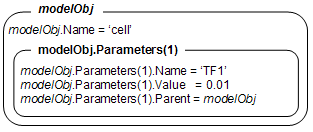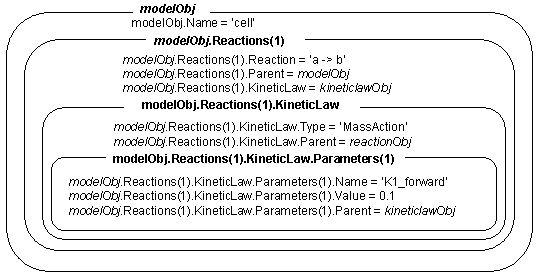Create parameter object and add to model or kinetic law object

## Syntax

```parameterObj = addparameter(Obj, 'NameValue') parameterObj = addparameter(Obj, 'NameValue', ValueValue) parameterObj = addparameter(...'PropertyName', PropertyValue...) ```

## Arguments

 `Obj` `Model object` or `kineticlaw object`. Enter a variable name for the object. `NameValue` Property for a parameter object. Enter a unique character vector. Since objects can use this property to reference a parameter, a parameter object must have a unique name at the level it is created. For example, a kinetic law object cannot contain two parameter objects named `kappa`. However, the model object that contains the kinetic law object can contain a parameter object named `kappa` along with the kinetic law object.For information on naming parameters, see `Name`. `ValueValue` Property for a parameter object. Enter a number.

## Description

```parameterObj = addparameter(Obj, 'NameValue') ```creates a parameter object and returns the object (`parameterObj`). In the parameter object, this method assigns a value (`NameValue`) to the property `Name`, assigns a value `1` to the property `Value`, and assigns the model or kinetic law object to the property `Parent`. In the model or kinetic law object, (`Obj`), this method assigns the parameter object to the property `Parameters`.

A parameter object defines an assignment that a model or a kinetic law can use. The scope of the parameter is defined by the parameter parent. If a parameter is defined with a kinetic law object, then only the kinetic law object and objects within the kinetic law object can use the parameter. If a parameter object is defined with a model object as its parent, then all objects within the model (including all rules, events and kinetic laws) can use the parameter.

```modelObj = sbiomodel('cell') parameterObj = addparameter(modelObj, 'TF1', 0.01) ``````modelObj = sbiomodel('cell') reactionObj = addreaction(modelObj, 'a -> b') kineticlawObj = addkineticlaw (reactionObj, 'MassAction') parameterObj = addparameter(kineticlawObj, 'K1_forward', 0.1) ``````parameterObj = addparameter(Obj, 'NameValue', ValueValue)``` creates a parameter object, assigns a value (`NameValue`) to the property `Name`, assigns the value (`ValueValue`) to the property `Value`, and assigns the `model object` or the `kineticlaw object` to the property `Parent`. In the model or kinetic law object (`Obj`), this method assigns the parameter object to the property `Parameters`, and returns the parameter object to a variable (`parameterObj`).

``` parameterObj = addparameter(...'PropertyName', PropertyValue...)``` defines optional property values. The name-value pairs can be in any format supported by the function `set`.

Scope of a parameter — A parameter can be scoped to either a model or a kinetic law.

• When a kinetic law searches for a parameter in its expression, it first looks in the parameter list of the kinetic law. If the parameter isn’t found there, it moves to the model that the kinetic law object is in and looks in the model parameter list. If the parameter isn’t found there, it moves to the model parent.

• When a rule searches for a parameter in its expression, it looks in the parameter list for the model. If the parameter isn’t found there, it moves to the model parent. A rule cannot use a parameter that is scoped to a kinetic law. So for a parameter to be used in both a reaction rate equation and a rule, the parameter should be scoped to a model.

Additional parameter object properties can be viewed with the `get` command. Additional parameter object properties can be modified with the `set` command. The parameters of `Obj` can be viewed with ```get(Obj, 'Parameters').```

A SimBiology® parameter object can be copied to a SimBiology model or kinetic law object with `copyobj`. A SimBiology parameter object can be removed from a SimBiology model or kinetic law object with `delete`.

## Method Summary

Methods for parameter objects

 copyobj Copy SimBiology object and its children delete Delete SimBiology object display Display summary of SimBiology object findUsages Find out how a species, parameter, or compartment is used in a model get Get SimBiology object properties move Move SimBiology species or parameter object to new parent rename Rename object and update expressions set Set SimBiology object properties

## Property Summary

Properties for parameter objects

 Constant Specify variable or constant species amount, parameter value, or compartment capacity ConstantValue Specify variable or constant parameter value Name Specify name of object Notes HTML text describing SimBiology object Parent Indicate parent object Tag Specify label for SimBiology object Type Display SimBiology object type Units Units for species amount, parameter value, compartment capacity, observable expression UserData Specify data to associate with object Value Value of species, compartment, or parameter object ValueUnits Parameter value units

## Example

1. Create a model object, and then add a reaction object.

```modelObj = sbiomodel ('my_model'); reactionObj = addreaction (modelObj, 'a + b -> c + d');```
2. Define a kinetic law for the reaction object.

`kineticlawObj = addkineticlaw(reactionObj, 'MassAction');`
3. Add a parameter and assign it to the kinetic law object (`kineticlawObj`); add another parameter and assign to the model object (`modelObj`).

```% Add parameter to kinetic law object parameterObj1 = addparameter (kineticlawObj, 'K1'); get (kineticlawObj, 'Parameters')```

MATLAB returns:

```SimBiology Parameter Array Index: Name: Value: ValueUnits: 1 K1 1 ```
```% Add parameter with value 0.9 to model object parameterObj1 = addparameter (modelObj, 'K2', 0.9); get (modelObj, 'Parameters')```
MATLAB returns:
```SimBiology Parameter Array Index: Name: Value: ValueUnits: 1 K2 1 ```

## Version History

Introduced in R2006a

expand all